# Heart of AlgebraPage 3

#### WATCH ALL SLIDES

Algebra is a prerequisite for advanced mathematics

Slide 16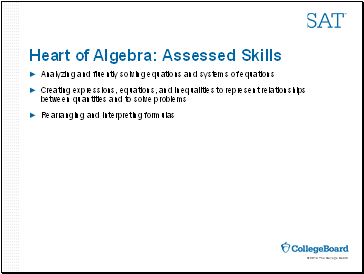## Heart of Algebra: Assessed Skills

Analyzing and fluently solving equations and systems of equations

Creating expressions, equations, and inequalities to represent relationships between quantities and to solve problems

Rearranging and interpreting formulas

Slide 17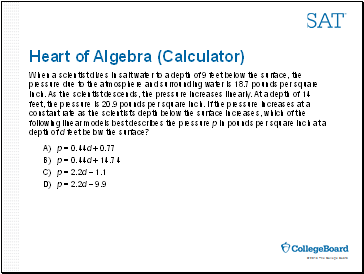## Heart of Algebra (Calculator)

When a scientist dives in salt water to a depth of 9 feet below the surface, the pressure due to the atmosphere and surrounding water is 18.7 pounds per square inch. As the scientist descends, the pressure increases linearly. At a depth of 14 feet, the pressure is 20.9 pounds per square inch. If the pressure increases at a constant rate as the scientist’s depth below the surface increases, which of the following linear models best describes the pressure p in pounds per square inch at a depth of d feet below the surface?

A) p = 0.44d + 0.77

B) p = 0.44d + 14.74

C) p = 2.2d – 1.1

D) p = 2.2d – 9.9

Slide 18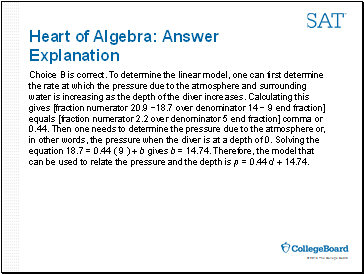Explanation

Choice B is correct. To determine the linear model, one can first determine the rate at which the pressure due to the atmosphere and surrounding water is increasing as the depth of the diver increases. Calculating this gives [fraction numerator 20.9 −18.7 over denominator 14 − 9 end fraction] equals [fraction numerator 2.2 over denominator 5 end fraction] comma or 0.44. Then one needs to determine the pressure due to the atmosphere or, in other words, the pressure when the diver is at a depth of 0. Solving the equation 18.7 = 0.44 ( 9 ) + b gives b = 14.74. Therefore, the model that can be used to relate the pressure and the depth is p = 0.44 d + 14.74.

Slide 19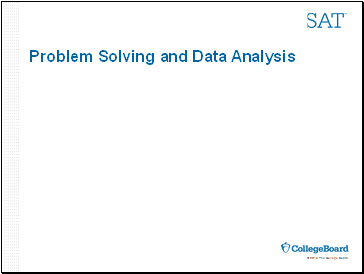## Problem Solving and Data Analysis

Slide 20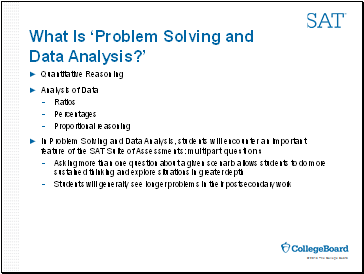What Is ‘Problem Solving and Data Analysis?’

Quantitative Reasoning

Analysis of Data

Ratios

Percentages

Proportional reasoning

In Problem Solving and Data Analysis, students will encounter an important feature of the SAT Suite of Assessments: multipart questions

Asking more than one question about a given scenario allows students to do more sustained thinking and explore situations in greater depth

Students will generally see longer problems in their postsecondary work

Slide 21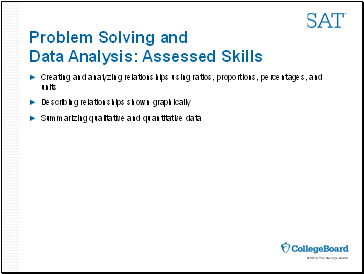Problem Solving and Data Analysis: Assessed Skills

Creating and analyzing relationships using ratios, proportions, percentages, and units

Go to page:
1  2  3  4  5  6  7